Next: Fitting Software Up: Analysis Previous: Data Fitting in the Time Domain

# Model for the Internal Field Distribution

The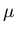SR data is fitted to a London model  that incorporates the effects of nonlocality though a kernel  Q(k), an extension described in Section 2.1. As mentioned in that section, the London model is inapplicable within a vortex core. Despite this the theory can yield the vortex lattice structure belonging to high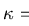superconductors, such as LuNi2B2C, under weak external fields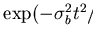, because in these situations the intervortex spacing greatly surpasses the diameter of a core . LuNi2B2C exhibits both square and triangular vortex structures according to the field  H applied, as discussed in Chapter 3. This nonlocal theory is chosen to fit theSR data since it accounts for an observed vortex lattice transformation between these two geometries in LuNi2B2C, and because the data are collected under low field () conditions.

Expanding  the BCS kernel Q(k), for weak currents J, to first order in the small term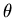leads to the internal spatial field profile B(r) of a nonlocal London model: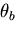(7.1)

where the co-ordinates (x, y, z) coincide with the crystal frame (a, b, c) and the external field  H is in the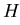direction. The average magnetic field inside the superconducting sample is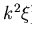. The constant co-efficients 0.0705 and 0.675 arise from the evaluation of Fermi surface averages of products of Fermi velocities according to LuNi2B2C band structure. The Gaussian cutoff factorin the sum over the reciprocal lattice vectors k of the vortex lattice compensates for the failure of the London approach within the core region. This model neglects vortex-vortex interactions. The parameter C reflects the strength of the nonlocal effects, and contains several poorly known factors.

The nonlocality parameter C varies theoretically with temperature T as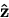(7.2)

where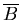is the nonlocality radius, on the order of the BCS coherence length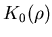. Figure 5.1 shows the numerically calculated behaviour of the penetration depth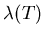, the nonlocality radiusand the nonlocality parameter C(T)as functions of temperature.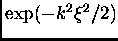The relation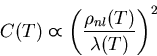(7.3)

where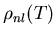are the Matsubara frequencies and n is a non-negative integer, gives the expected temperature dependence of the penetration depth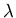in the clean limit. Solving  the equation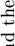(7.4)

determines the BCS energy gap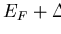, whereis the Debye energy, N(0) is the density of states at the Fermi level for electrons of one spin orientation, and V describes the strength of the interaction potential for scattering a Cooper pair. Evaluating the above integral at temperature T = Tc reveals the constant N(0)V to be

 (7.5)

whereis Euler's constant. The calculations plotted in Figure 5.1 assume a critical temperature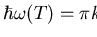and a Debye temperature, values appropriate for LuNi2B2C. The expression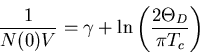(7.6)

generates the clean limit behaviour of the nonlocality radiusshown in this figure. The above sums over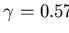converge by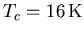. Combining the temperature dependence of the penetration depthand the nonlocality radiusaccording to (5.3) produces the temperature variation of the internal field model parameter C, displayed in Figure 5.1. The next section describes the software utilised to fit this internal field model to the experimental data.Next: Fitting Software Up: Analysis Previous: Data Fitting in the Time Domain
Jess H. Brewer
2001-10-31